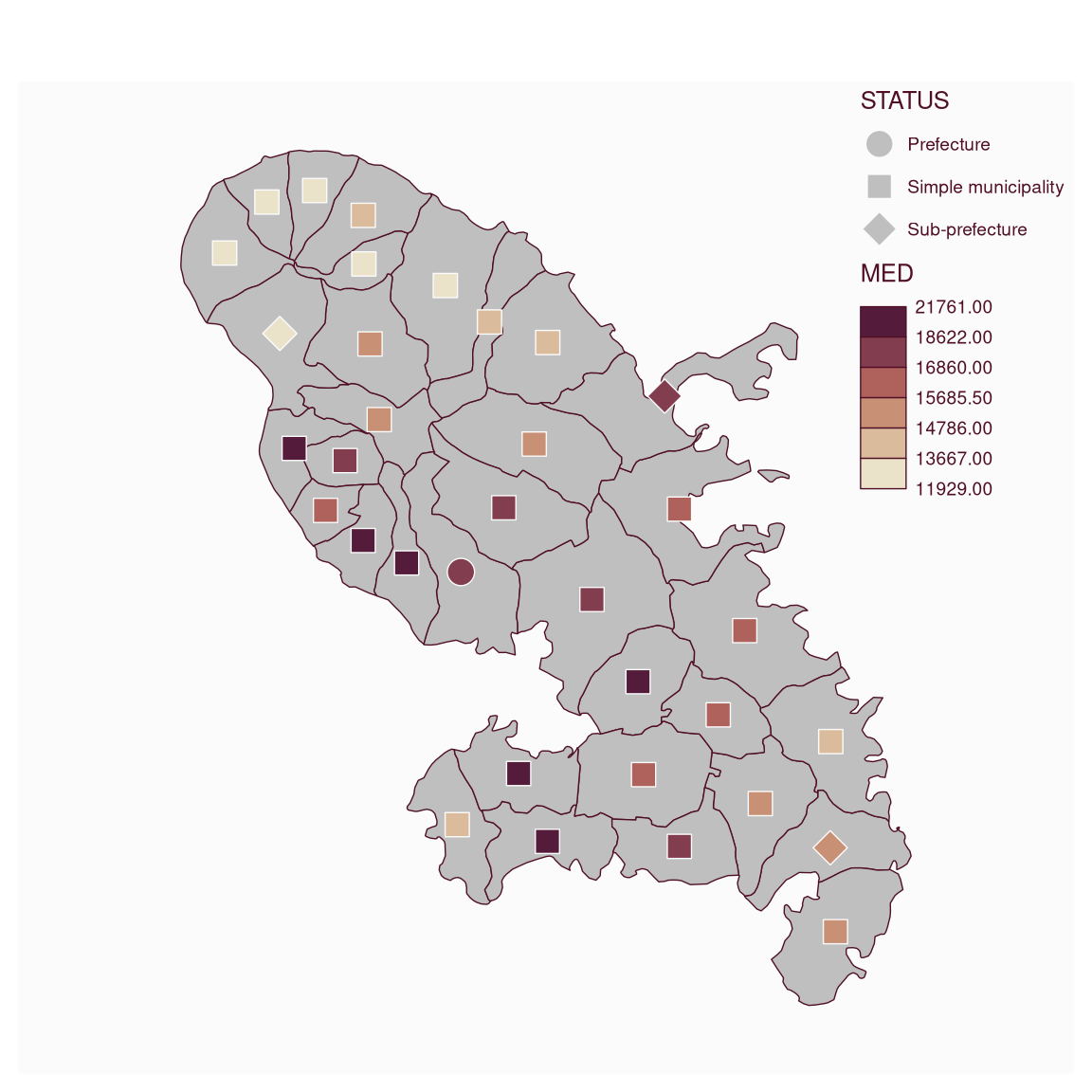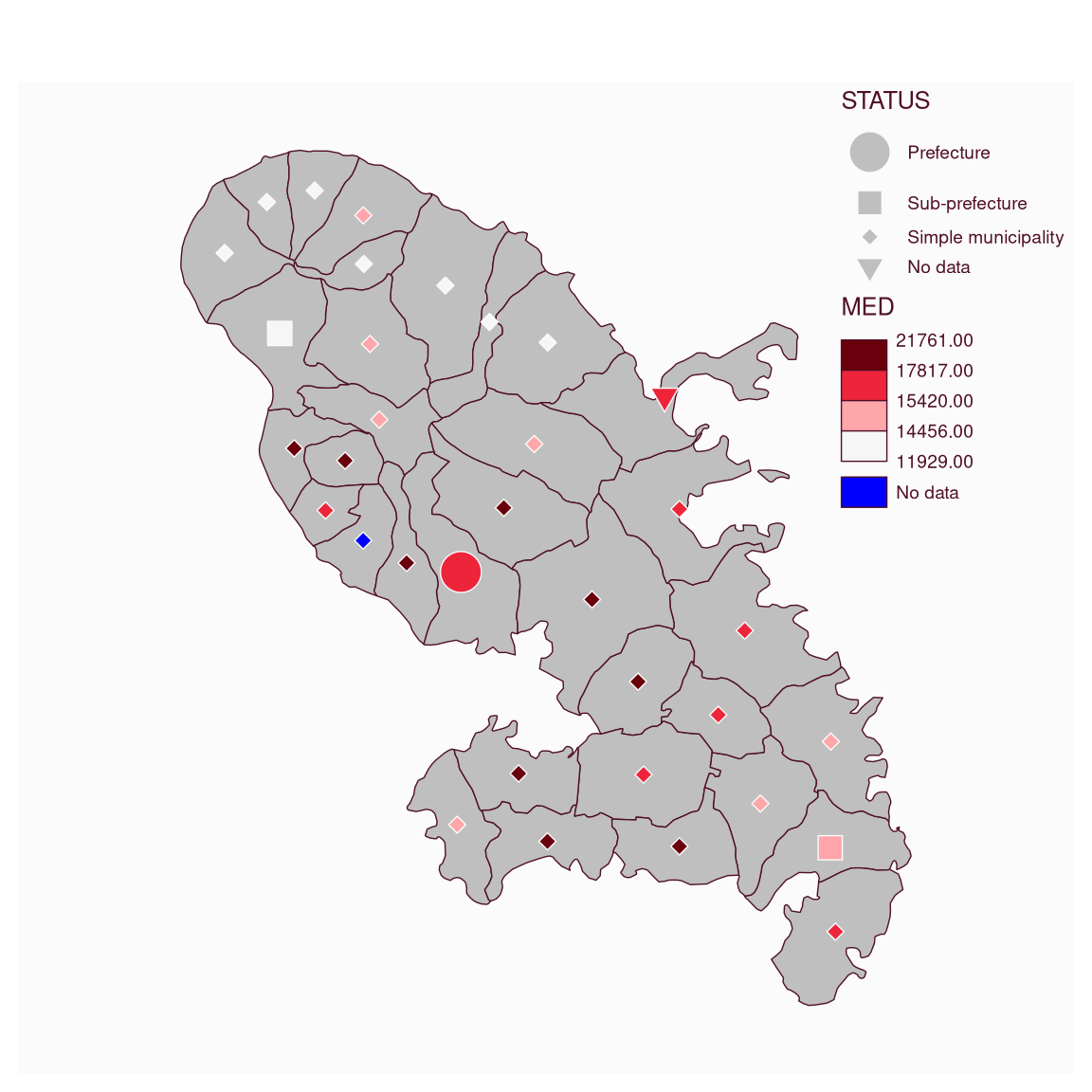Plot symbols with colors based on a quantitative data classification.

mf_symb_choro(
x,
var,
pal = "Mint",
alpha = 1,
breaks = "quantile",
nbreaks,
border,
pch,
cex = 1,
lwd = 0.7,
pch_na = 4,
cex_na = 1,
col_na = "white",
val_order,
leg_pos = mf_get_leg_pos(x, 2),
leg_title = var,
leg_title_cex = c(0.8, 0.8),
leg_val_cex = c(0.6, 0.6),
leg_val_rnd = 2,
leg_no_data = c("No data", "No data"),
leg_frame = c(FALSE, FALSE),
)

## Arguments

x

object of class sf

var

name(s) of the variable(s) to plot

pal

a set of colors or a palette name (from hcl.colors)

alpha

if pal is a hcl.colors palette name, the alpha-transparency level in the range [0,1]

breaks

either a numeric vector with the actual breaks, or a classification method name (see mf_get_breaks and Details)

nbreaks

number of classes

border

border color

pch

pch (point type) for symbols

cex

cex (point size) for symbols

lwd

border width

pch_na

pch (point type) for NA values

cex_na

cex (point size) for NA values

col_na

color for missing values

val_order

values order, a character vector that matches var modalities

leg_pos

position of the legend, two of 'topleft', 'top','topright', 'right','bottomright', 'bottom', 'bottomleft', 'left' or a vector of two coordinates in map units (c(x, y)). leg_pos argument can be c('position', 'position'), c('position', x2, y2), c(x1,y1, 'position') or c(x1, y1, x2, y2). Use NA to avoid plotting the legend, use 'interactive' to choose thelegend position interactively.

leg_title

legend title

leg_title_cex

size of the legend title

leg_val_cex

size of the values in the legend

leg_val_rnd

number of decimal places of the values in the legend

leg_no_data

label for missing values

leg_frame

whether to add a frame to the legend (TRUE) or not (FALSE)

whether to add the layer to an existing plot (TRUE) or not (FALSE)

## Value

x is (invisibly) returned.

## Details

Breaks defined by a numeric vector or a classification method are left-closed: breaks defined by c(2, 5, 10, 15, 20) will be mapped as [2 - 5[, [5 - 10[, [10 - 15[, [15 - 20]. The "jenks" method is an exception and has to be right-closed. Jenks breaks computed as c(2, 5, 10, 15, 20) will be mapped as [2 - 5], ]5 - 10], ]10 - 15], ]15 - 20].

## Examples

mtq <- mf_get_mtq()
mf_map(mtq)
mf_symb_choro(mtq, c("STATUS", "MED"))mf_map(mtq)
mtq$STATUS <- NA mtq$MED <- NA
mf_symb_choro(mtq, c("STATUS", "MED"),
pal = "Reds 3", breaks = "quantile", nbreaks = 4,
pch = 21:23, cex = c(3, 2, 1),
pch_na = 25, cex_na = 1.5, col_na = "blue",
val_order = c(
"Prefecture",
"Sub-prefecture",
"Simple municipality"
)
)